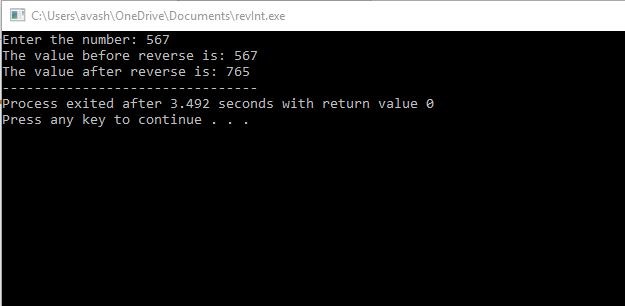# Question & Answer: Write a C++ program that defines a function int reverseInt(int n) that takes as an argument a positive integ…..

Write a C++ program that defines a function int reverseInt(int n) that takes as an argument a positive integer and returns an integer with digits in reverse order. Print n before and after reversing the digits. Your output should have the same format and should work for any integer a user enters.

Desired Output

Don't use plagiarized sources. Get Your Custom Essay on
Question & Answer: Write a C++ program that defines a function int reverseInt(int n) that takes as an argument a positive integ…..
GET AN ESSAY WRITTEN FOR YOU FROM AS LOW AS \$13/PAGE

The value before reverse is: 425

The value after reverse is: 524

In the C++ code below, we have the reverseInt() function that takes in an integer, num as an argument.

It reverses the integer and returns the reversed integer.

It has two integers, rev and rem that store the reverse and the remainder values.

It works like this: let num be 425.

Then remainder = num%10 that is = 5

and rev = rev*10 + rem that is 0*10 + 5 = 5

then num = num/10 gives num = 42

again loop iterates, as num>0

now, rem = 2, rev = 52 and num = 4

again loop iterates as num>0

now, rem = 4, rev = 524 and num = 0

Loop terminates. And 5524 is returned.

CODE:

#include<iostream>

using namespace std;

int reverseInt(int num)

{

int rem, rev = 0;

while(num > 0)

{

rem = num % 10;

rev = rev * 10 + rem;

num = num/ 10;

}

return rev;

}

int main()

{

int num;

cout<<“Enter the number: “;

cin>>num;

cout<<“The value before reverse is: “<<num;

cout<<“nThe value after reverse is: “<<reverseInt(num);

return 0;

}

Output: